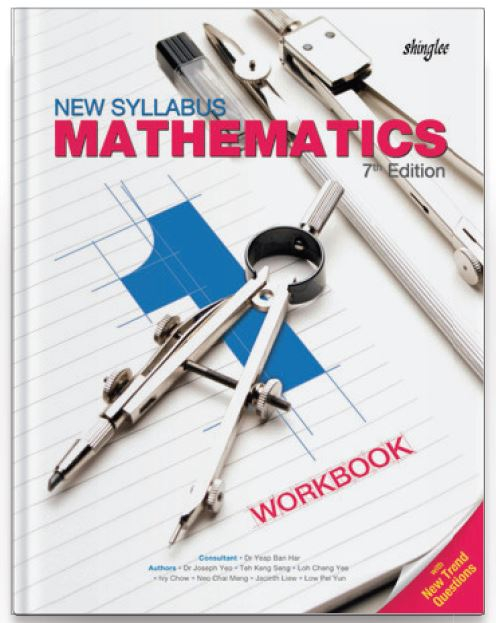Our prices are denominated in USD.

We ship internationally!# New Syllabus Mathematics Workbook 1 (7th Ed)

US\$11.25
Not Available

New Syllabus Mathematics is the most widely-adopted series of secondary mathematics textbooks in Singapore. Following the three-part lesson approach, pupils begin with an anchor task, followed by a guided practice, before concluding with an independent practice. New concepts are introduced and built upon in the textbooks. Well-structured exercises developed in
accordance to the lesson objectives are found in the workbooks.

This is a 4-year course in preparation for Cambridge O-level.

This is a 4-year course.

For Secondary 1/ Grade 7 / Year 7

1. Primes, Higher Common Factor and Lowest Common Multiple
Square and Cube Roots

2. Integers, Rational Numbers and Real Numbers
Four Operations of Negative Numbers

3. Approximation and Estimation
Significant Numbers, Rounding and Estimation

4. Basic Algebra and Algebraic Manipulation
Expansion, Factorisation and Simplification of Linear Expressions and with Fractional Coefficients

5. Linear Equations and Simple Inequalities

6. Functions and Linear Graphs
Gradient of Straight Line and Applications of Linear Graph in Real-World Contexts

7. Number Patterns
Number Sequences, General Term

8. Percentage
Percentage Change, Reverse Percentage and in Practical Situations

9. Ratio, Rate and Speed

10. Basic Geometry
Points, Lines, Planes, Angles and Angles Related to  Parallel Lines

12. Geometrical Constructions
Perpendicular and Angle Bisectors and Constructions of Triangles and Quadrilaterals

13. Perimeter and Area of Plane Figures
Basic Plane Figures, Parallelograms and Trapeziums

14. Volume and Surface Area of Prisms and Cylinders
Cubes, Cuboids, Prisms, Cylinders and Composite Solids

15. Statistical Data Handling
Pictograms, Bar Graphs, Pie Charts, Line Graphs